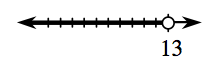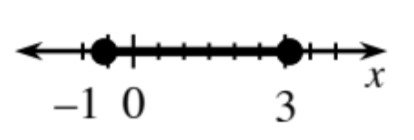### Home > CCA2 > Chapter 4 > Lesson 4.2.3 > Problem4-94

4-94.

Solve each of the following inequalities. Express the solutions algebraically and on a number line.

1. $3(x+2)>4x−7$

$3(x+2)=4x−7\\3x+6=4x−7\\13=x$

Plot the boundary point. Should it be shaded or not? Test values on either side of $13$. Which side makes it true?

$x<13$1. $3x^2−4x+2\le x^2+x+6$

See part (a). Rearrange the equation so that it equals zero.

The equation can't be factored. Use the Quadratic Formula.

Plot the boundary points. Test a point between them. Does it make the inequality true? If so, shade between.
If not, test points on the outside.

$\frac{5-\sqrt{57}}{4}\le x\le\frac{5+\sqrt{57}}{4}$

or

$0.637\le x\le3.137$